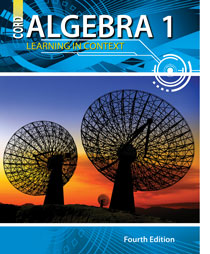# Algebra 1 - 4th Edition

## Chapter 11: Statistics

Some links are repeated for use with more than one lesson.

### These sites apply to the entire chapter.

http://www.freemathhelp.com/Lessons/Algebra_2_Probability_and_Statistics_BB.htm
This page contains a video tutorial with notes covering an introduction to probability and statistics.

http://www.robertniles.com/stats/
This site defines the different components of statistics.

### 11.1 Measures of Central Tendency

This page contains word problems that practice the measures of central tendency.

### 11.2 Frequency Distributions and Histograms

http://www.sparknotes.com/math/algebra1/graphingdata/section2.rhtml
This page demonstrates how to make a frequency distribution and histogram from some sample data.

http://www.mathsisfun.com/data/frequency-distribution.html

### 11.3 Dot Plots

http://staff.argyll.epsb.ca/jreed/math9/strand4/scatterPlot.htm
This site defines scatter plots and lines of best fit and has interactive graph applets on which to plot points.

### 11.4 Box Plots

http://www.purplemath.com/modules/boxwhisk.htm
This site explains quartiles and box-and-whisker plots through text and graphs.

This page explains box-and-whisker plots and their quartiles and the special case.

### 11.5 Measures of Dispersion

http://simon.cs.vt.edu/SoSci/converted/Dispersion_I/activity.html
Java applets that interactively teach about measures of dispersion.

http://www.mathsisfun.com/data/standard-deviation.html